Thanksgiving SALE! Get Math Mammoth and Make It Real Learning digital downloads at 30% off — right here at the MathMammoth.com website. Use coupon code THANKS30 at checkout. Offer valid through December 2, 2023.

^
You are here: HomeFAQs → FAQ: editions

# Changes in the different editions of Math Mammoth curriculum

This document explains the main changes between the different editions of Math Mammoth curriculum (Light Blue Series).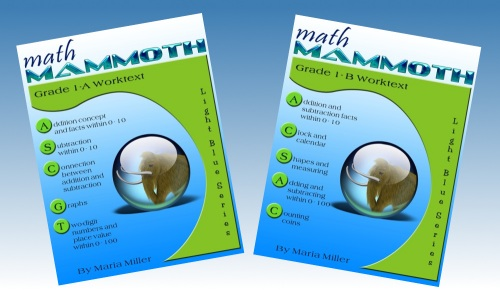There have been two editions for grade 1:

• The 2007 (pre-common-core) edition
• The 2012 edition
Changes in the 2012 edition
• What used to be chapter 4 (Place Value) switched places with Chapter 3 (Addition and Subtraction Facts within 0-18).
• The topics of rounding, even & odd numbers, and parenthesis were moved to grades 2 and 3.
• There are many new lessons about adding and subtracting within 20, and adding and subtracting two-digit numbers without regrouping, explaining basic mental math principles.
• The lesson "Exploring Measuring" is split into two lessons (Measuring Length and Exploring Measuring). Both lessons are expanded from what they were before.
• Geometry section was expanded and changed. It now has more material about shapes.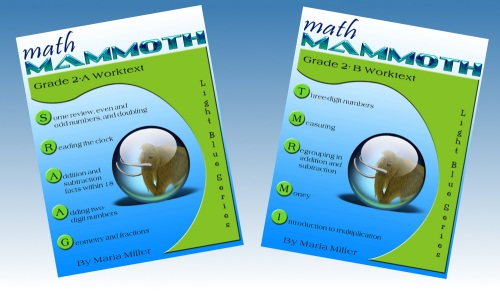There have been two editions for grade 2:

• The 2007 (pre-common-core) edition;
• The 2012 edition
Changes in the 2012 edition
• The topics of rounding and finding 1/4 of a number were taken off (moved to grade 3).
• The topics of regrouping in addition and regrouping in subtraction are now split so that 2-A contains regrouping in addition, and 2-B contains regrouping in subtraction.
• There is now more explicit (more scaffolded) instruction about adding four single-digit numbers, and then adding four two-digit numbers in columns (in 2-A).
• Regrouping in subtraction with three-digit numbers is also scaffolded more (the progression of the concept presentation is by smaller steps) (in 2-B). Regrouping with zero tens is still left for third grade, as before (such as in 506 − 358).
• There are more word problems in general. As before the change, the curriculum still has lots of instruction about mental math in addition and subtraction.
• Geometry chapter concentrates on naming basic shapes such as triangles, quadrilaterals, pentagons, and hexagons, putting shapes together to form new ones, dividing shapes into new ones, and geometric patterns. The topics of right angle and parallel lines were taken off as they are not in the CCS for grade 2.
• In measuring, the topic of volume is moved to third grade in accordance to Common Core Standards. The topics in 2nd grade include inches, half-inches, centimeters, feet, miles, meters, kilometers, and weight in pounds and in kilograms. Compared to the earlier edition, I have added line plots, exercises about measuring objects both in inches and in centimeters, estimating lengths, and finding how much longer one thing is than another.
• The topics of clock, place value with 3-digit numbers, graphs, and introduction to multiplication didn't have any significant conceptual changes (just cosmetic ones).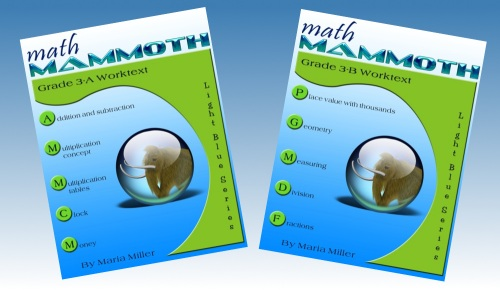There have been three editions for grade 3:

• The 2007 (pre-common-core) edition
• The 2012 edition
Changes in the 2012 edition
• The geometry chapter has been pretty much redone. Now, it concentrates on the concepts of area and perimeter (according to Common Core standards). Before, I did have one lesson on area, but also other concepts, such as right angles and parallel lines. Those will be moved to 4th grade.
• In measuring, I have moved most of the content that dealt with conversions between the measuring units to 4th grade. I did leave some content and easy exercises about it, though, as I feel 3rd graders are able to do such. I also reorganized some of the material here and added new measuring activities.
• In fractions, I added the topics of fractions on a number line, equivalent fractions, and comparing fractions, and removed the topic of adding and subtracting like fractions.
• The topic of decimal numbers has been removed from this grade.
• The 2018 edition
Changes in the 2018 edition
• The lessons on elapsed time were revised.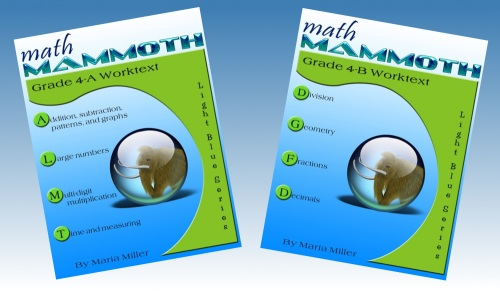There have been four editions for grade 4:

• The 2008 (pre-common-core) edition
• The 2012 edition
Changes in the 2012 edition

The main changes are that some topics were taken off and moved to 5th grade, and several topics were added in. Other than that, the basic framework and conceptual development did not change substantially. Of course, I edited the lessons to make them better, added more space or grids for calculations, etc. so there were many cosmetic changes.

• Numbers beyond 1,000,000
• multiplying a 3-digit number by a 2-digit number
• 2-digit divisor in long division
• multiplying decimals by whole numbers
• circles
• volume
• In measuring, the problems about conversions between units are easier, and the more challenging conversions will be in 5th grade.

• divisibility
• prime numbers
• finding factors of a given number
• multiplying fractions by whole numbers
• adding a fraction with tenths and another with hundredths (such as 3/10 + 8/100)
• Line symmetry
• The 2018 edition
Changes in the 2018 edition
• The lessons on elapsed time and some lessons in the geometry chapter on angles were revised.
• The 2020 edition
Changes in the 2020 edition
• Several lessons were completely revised (on the topics of order of operations, multiplying money amounts, adding fractions, mixed numbers, subtracting mixed numbers, using decimals with measuring units).
• Some topics are now covered in two lessons instead of one and the lessons were revised (multiplying in parts, the remainder, review of area of rectangles).
• The lesson "Finding Fractional Parts with Division" was moved into a different place within the chapter.
• There are minor changes in individual exercises and text throughout.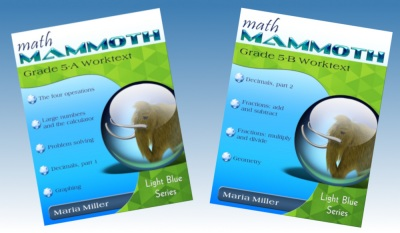• The 2009 (pre-common-core) edition
• The 2013 edition
Changes in the 2013 edition
• The biggest changes in grade 5 are that the two optional chapters (percent and integers) have been removed, and the topics in geometry chapter are different. However, overall, the structure and contents of this grade is very similar to the old. Grade 5 in Math Mammoth slightly exceeds the Common Core Standards.
• Chapter 1 starts out like the old version, with some review topics and multi-digit multiplication. There is now more focus and practice problems on long division with two-digit divisors. One big change is that the lessons on problem solving and equations were moved to their own chapter (chapter 3 in the revised version). Another is that I have now included the topics of divisibility, factors and primes, and prime factorization (used to be in the old version grade 6).
• Chapter 2 (large numbers) is also very similar to the old version. The lesson A Little Bit of Millions was added (it used to be in 4th grade).
• Chapter 3 is about problem solving and simple equations (originally these lessons were in the 1st chapter). This chapter exceeds the CCS.
• The chapter about decimals is very similar to the old version in its contents. The topic of writing decimals in expanded form was added to one of the lessons. Other changes are cosmetic - improved layout, images, scaffolding of concepts, and order of presentation (in some lessons).
• The chapter on graphing and statistics is very similar to the old version. I added one more lesson on patterns in the coordinate grid. This chapter is now Chapter 5, and is moved to the part B.

The chapter exceeds Common Core standards for 5th grade (the lessons on line graphs, histograms, analyzing graphs, mean, and mode), because the CCS do not include statistics topics in 5th grade. However, I feel students will need this as a background before the statistics topics of 6th grade, which are somewhat advanced. For example, in 6th grade students will study interquartile range and/or mean absolute deviation, and will need to relate the choice of measures of center and variability to the shape of the data distribution and the context in which the data were gathered. I do not feel that you can read Common Core Standards as "include this and nothing else", because the standards are not all-inclusive: there are many topics they do not explicitly mention. In my opinion, for students to be able to make sense of mean absolute deviation and relate the choice of measures of center & variability to the shape of the data distribution in 6th grade, they need to analyze and study statistical graphs in easier manners in 5th grade.
• Chapter 6 is fraction addition and subtraction. It is, again, very similar to the earlier version in its contents. I have added a few new problems about how to spot calculation errors using "fraction number sensen" and about line plots.
• Chapter 7 is fraction multiplication and division. It is similar to the earlier version, but has some changes as well. The lesson Fraction Multiplication and Area is expanded to include problems where the student extends the sides of the given rectangle to get a square unit, and then write a multiplication for the area of the original rectangle. There are two new lesson Multiplication as Scaling/Resizing and Fractions are Divisions. Line plots are included in several of the lessons.

Division of fractions is only dealt in these special cases: sharing divisions (such as (4/6) ÷ 2), dividing unit fractions by whole numbers (for example (1/5) ÷ 3) and dividing whole numbers by unit fractions (such as 5 ÷ (1/3)). These types of divisions can be solved with mental reasoning, without using the "rule." The rest is left for 6th grade.

• Chapter 8 is geometry, and its topics are different from the older version. Now, it includes a review of angles, a review of area and perimeter, drawing circles, classifying triangles, classifying quadrilaterals, and volume of rectangular prisms. It used to include many lessons about the area of polygons, which will now move to 6th grade.
• The 2020 edition
Changes in the 2020 edition
• The lessons on the calculator have been completely redone.
• The long chapter on decimals was split into two, and the chapter on graphing and statistics was placed in between. The latter chapter on decimals reviews some of the topics from the first. All the lessons about decimals were revised, and many were completely rewritten to have better scaffolding of the concepts.
• Several lessons on other topics (pan balance and equations , histograms, multiply fractions by whole numbers ) were completely revised.
• Some topics are now covered in two lessons instead of one and the lessons were completely revised (adding and subtracting mixed numbers, multiplying fractions by fractions, volume of rectangular prisms).
• Division of fractions is now approached differently. There is a new lesson about the shortcut for fraction division, and the existing lessons on fraction division were completely redone.
• The topic of ratios (covered in one lesson) was removed from this grade (it is covered in 6th and 7th).
• There are minor changes in individual exercises and text throughout.
• The 2023 edition
• Changes in the 2023 edition
• The pacing has been standardized so that each lesson is now suggested to take either 1 or 2 days.
• Several lessons are marked as optional, to make it easier to plan (e.g. Long Division as Repeated Subtraction, Star Polygons).
• Several longer lessons, especially in chapter 1, were reorganized into two separate lessons, and rewritten.
• Several lessons were rewritten completely, especially in chapters 1, 2, 5, and 9
• Chapter 5 (on statistical graphs) is now more focused, with fewer topics. The main topics are: the coordinate grid, patterns in the coordinate grid, and line graphs, and additionally we also study average (mean).
• In chapter 9 (geometry), the lessons on classifying triangles and quadrilaterals have been rewritten. The topic of circles was removed, and the lesson on star polygons marked as optional. Plus there were a few other changes to create a more focused presentation of the geometry topics for this level.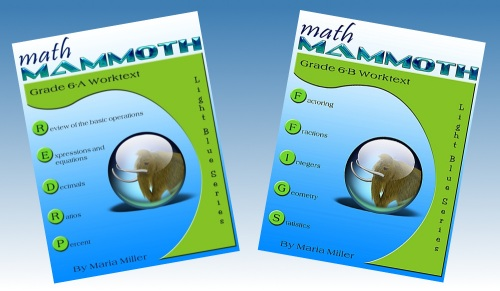There have been three editions for grade 6:

• The 2010 (pre-common-core) edition;
• The 2014 edition
Changes in the 2014 edition

In grade 6, there are several substantial changes. In Part A, the first chapter (review of the four operations) is similar to the old, but the rest of 6th grade has lots of changes.

Part A now has more coverage of algebraic concepts (evaluating and simplifying expressions, solving equations, inequalities) than before (chapter 2). The chapter on decimal arithmetic is fairly similar to the old version (chapter 3), but the topics of scientific notation and repeated decimals are moved to 7th. There is now more emphasis on ratios and rates (chapter 4). Percent lessons (chapter 5) are pretty much the same as before, but the topics of interest and percent of change are moved to 7th grade.

In part B, chapter 6 is about number theory, and is similar to the old version, but has a more detailed coverage of the greatest common factor and the least common multiple. In fractions (chapter 7), there is less review of old topics, and more emphasis on division of fractions and lessons on problem solving. Integers chapter is similar (chapter 8), but will omit multiplication and division of integers. Geometry topics (chapter 9) are changing nearly completely and will now emphasize area of polygons and surface area & nets.

Chapter 10 is statistics. It contains many of the same lessons and problems as the old, but has some new emphases, such as: students find the shape of distribution and determine which measure of center and measure of variability is the best to use based on the distribution. The topic of probability is moved to 7th.

• area of triangles and of polygons
• volume of rectangular prisms with fractional edge lengths
• writing, evaluating, and simplifying expressions
• solving simple linear equations
• inequalities
• a more detailed coverage of GCF and LCM
• a more detailed coverage of ratios and rates
• statistics: shape of distributions, mean absolute deviation

Topics moving to 7th grade that used to be in 6th in the old version:

• proportions
• scientific notation and repeated decimals
• percent of change and interest
• multiplication and division of integers
• most geometry topics
• probability
• The 2022 edition
Changes in the 2022 edition
• In this edition, the statistics chapter has been nearly completely rewritten. It now has more focus on understanding and describing distributions in general. The concept of mean absolute deviation now has its own lesson.
• In chapter 2, several lessons have been rewritten and some new lessons added, for better scaffolding of algebraic concepts.
• Chapters 6 (prime numbers, GCF, LCM), 7 (fractions), and 9 (geometry) also have had major improvements in the presentation and/or ordering of the concepts.
• The rest of the chapters have had only minor changes, such as in individual exercises.

There is only one edition for grade 7, from 2014 (for part A) and 2015 (part B).

WAIT!

Receive my monthly collection of math tips & resources directly in your inbox — and get a FREE Math Mammoth book!You can unsubscribe at any time.

### Math Mammoth Tour

Confused about the different options? Take a virtual email tour around Math Mammoth! You'll receive:

An initial email to download your GIFT of over 400 free worksheets and sample pages from my books. Six other "TOURSTOP" emails that explain the important things and commonly asked questions concerning Math Mammoth curriculum. (Find out the differences between all these different-colored series!)

This way, you'll have time to digest the information over one or two weeks, plus an opportunity to ask me personally about the curriculum.
A monthly collection of math teaching tips & Math Mammoth updates (unsubscribe any time)### "Mini" Math Teaching Course

This is a little "virtual" 2-week course, where you will receive emails on important topics on teaching math, including:

- How to help a student who is behind
- Troubles with word problems
- Teaching multiplication tables
- Why fractions are so difficult
- The value of mistakes
- Should you use timed tests
- And more!

A GIFT of over 400 free worksheets and sample pages from my books right in the very beginning.A monthly collection of math teaching tips & Math Mammoth updates (unsubscribe any time)
Enter your email to receive math teaching tips, resources, Math Mammoth news & sales, humor, and more! I tend to send out these tips about once monthly, near the beginning of the month, but occasionally you may hear from me twice per month (and sometimes less often).• A GIFT of over 400 free worksheets and sample pages from my books.